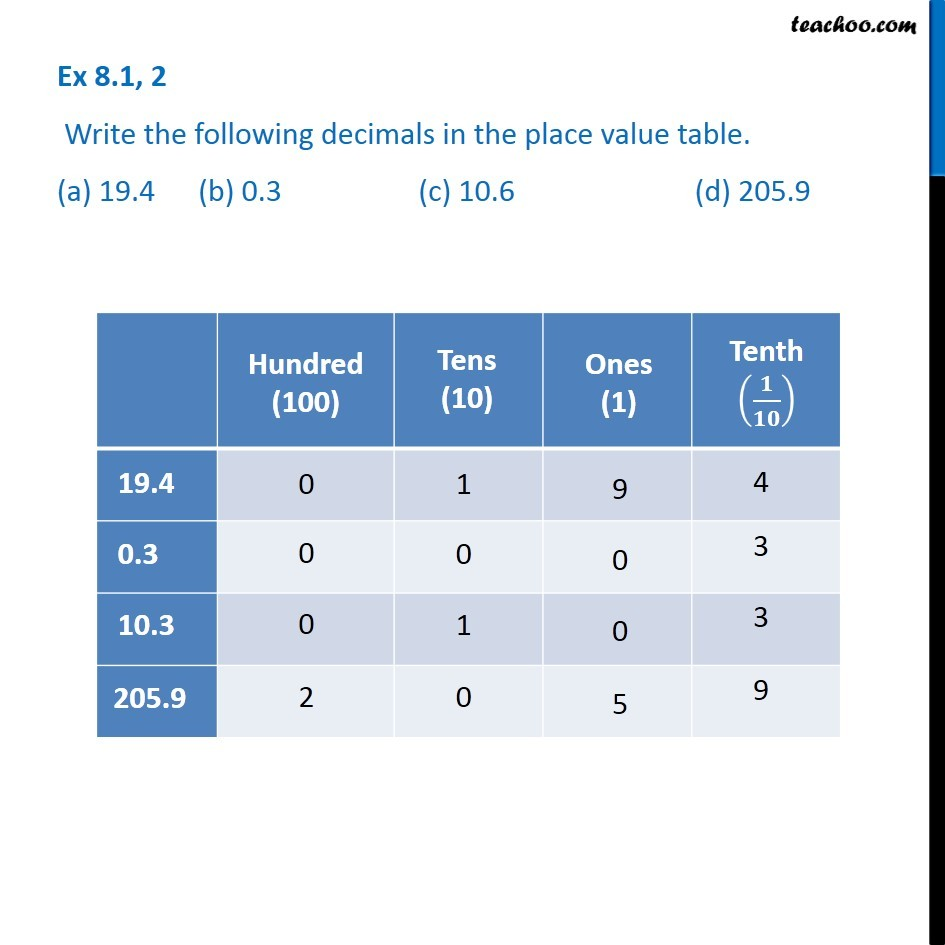Place Value Table

Chapter 8 Class 6 Decimals
Serial order wiseLearn in your speed, with individual attention - Teachoo Maths 1-on-1 Class

### Transcript

Question 2 - Chapter 8 Class 6 Decimals Write the following decimals in the place value table. (a) 19.4 (b) 0.3 (c) 10.6 (d) 205.9 For (a) Hundred (100) = 0 Tens (10) = 1 Ones (1) = 9 Tenths (1/10) = 4 For (b) Hundred (100) = 0 Tens (10) = 0 Ones (1) = 0 Tenths (1/10) = 3 For (c) Hundred (100) = 0 Tens (10) = 1 Ones (1) = 0 Tenths (1/10) = 3 For (d) Hundred (100) = 2 Tens (10) = 0 Ones (1) = 5 Tenths (1/10) = 9